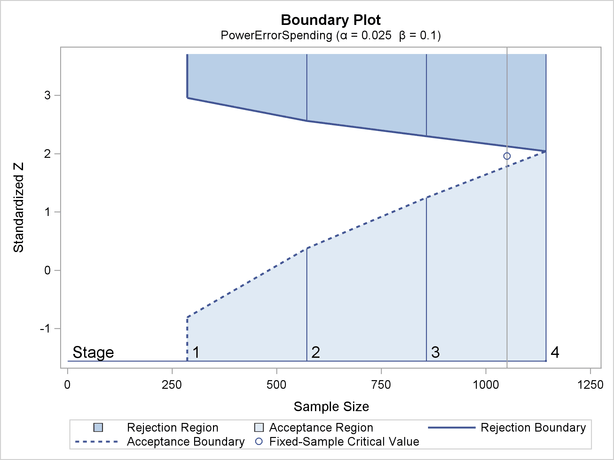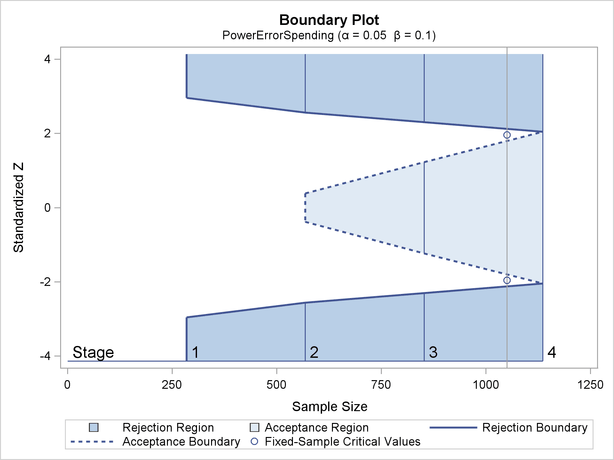Boundary Variables

The boundaries created in group sequential trials depend on the type of the alternative hypothesis and the early stopping criterion. Table 81.2 shows the boundaries created with various design specifications.

 Specifications Boundary Variables Alternative Lower Upper Hypothesis Early Stopping Alpha Beta Beta Alpha Lower AcceptX RejectX Accept/RejectX X Upper AcceptX RejectX Accept/RejectX X Two-sided AcceptX X RejectX X Accept/RejectX X X X

Up to four different boundaries can be generated in a group sequential design:

• the upperboundary, used to reject the null hypothesis in favor of an upper alternative hypothesis

• the upperboundary, used to accept the null hypothesis with an upper alternative hypothesis

• the lowerboundary, used to accept the null hypothesis with a lower alternative hypothesis

• the lowerboundary, used to reject the null hypothesis in favor of a lower alternative hypothesis

For a two-sided design, the null hypothesis is accepted only if both the null hypothesis is accepted with an upper alternative hypothesis and the null hypothesis is accepted with a lower alternative hypothesis.

For a one-sided design with a lower alternative, only the lower boundaries are created. Similarly, for a one-sided design with an upper alternative, only the upper boundaries are created. For example, Figure 81.19 shows the boundary plot for a one-sided test with an upper alternative.

Figure 81.19 Boundary Plot for One-Sided TestFigure 81.19 corresponds to a one-sided sequential design with early stopping to reject or accept the null hypothesis. For a sequential test with early stopping only to reject the null hypothesis, there are no acceptance boundary values at interim stages. The acceptance boundary value and its associated acceptance region are displayed only at the final stage. Similarly, for a sequential test with early stopping only to accept the null hypothesis, there are no rejection boundary values at interim stages. The rejection boundary value and its associated rejection region are displayed only at the final stage.

For a two-sided design, both the lower and upper boundaries are created. For a design with early stopping to reject the null hypothesis,boundaries are created. Similarly, for a design with early stopping to accept the null hypothesis,boundaries are created. For a design with early stopping to accept or reject the null hypothesis, both theandboundaries are created.

For example, Figure 81.20 shows the boundary plot for a two-sided test.

Figure 81.20 Boundary Plot for Two-Sided TestFigure 81.20 corresponds to a two-sided sequential design with early stopping to reject or accept the null hypothesis. For a sequential test with early stopping only to reject the null hypothesis, there are no acceptance boundary values at interim stages. The acceptance boundary value and its associated acceptance region are displayed only at the final stage. Similarly, for a sequential test with early stopping only to accept the null hypothesis, there are no rejection boundary values at interim stages. The rejection boundary value and its associated rejection region are displayed only at the final stage.